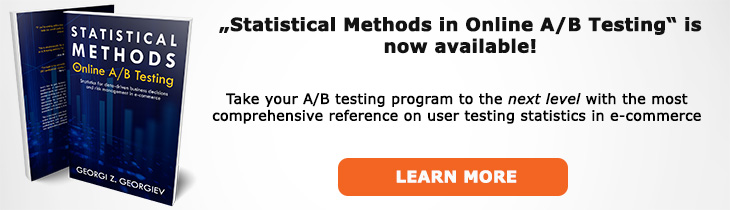# What does "T-test" mean?

Definition of T-test in the context of A/B testing (online controlled experiments).

## What is a T-test?

A T-Test (a.k.a. Student's T-test) is a statistical test which is based on the assumption of a T distribution of the parameter of interest and results in a t Score. Many classic statistical tests can operate under this assumption and produce results from a T distribution with T-distribution with n-k degrees of freedom where n is the total sample size and k is the number of test groups in an A/B test. The outcome of a T-test is a t score which can be transformed to a p-value by calculating the cumulative distribution function for the respective T-distribution.

A T-Test is usually performed when the population standard deviation (Σ) is unknown and the sample size is not large enough to invoke the Central Limit Theorem. This is rarely the case in A/B testing where the typical key performance indicator is an average (mean) and the sample size is usually in the thousands. The T and Z distribution (see Z-Test) are almost identical for sample sizes above 30 (30 degrees of freedom or above) but the the T distribution remains more robust.

A T-test can be paired or unpaired. An unpaired test is most often applicable in an A/B test scenario where we observe independent observations. If, however, we start with a certain group of users and measure a parameter before the intervention and then after it we would use a paired test in order to account for the presence of dependence. The paired test is a more powerful (as in statistical power) test and would result in a lower p-value compared to an unpaired test on the same data (which would be, of course, inadequate vis-a-vis the actual statistical model).

Like this glossary entry? For an in-depth and comprehensive reading on A/B testing stats, check out the book "Statistical Methods in Online A/B Testing" by the author of this glossary, Georgi Georgiev.## Glossary Index by Letter

Select a letter to see all A/B testing terms starting with that letter or visit the Glossary homepage to see all.